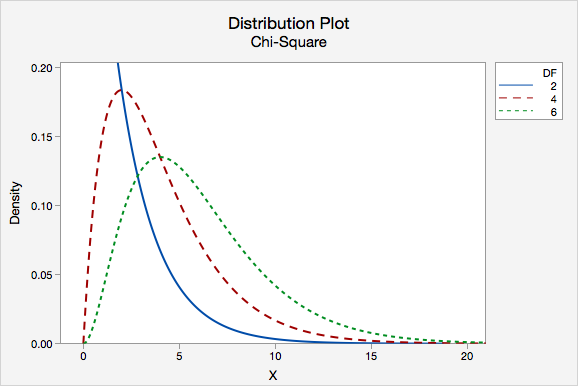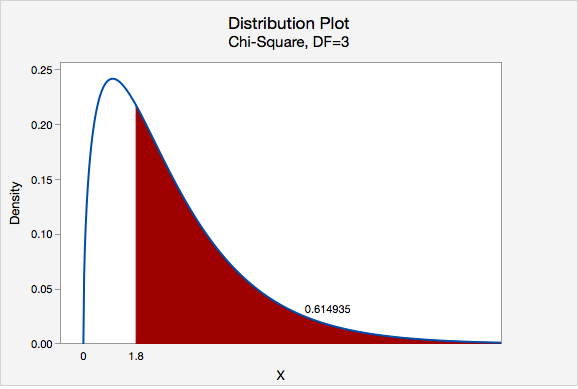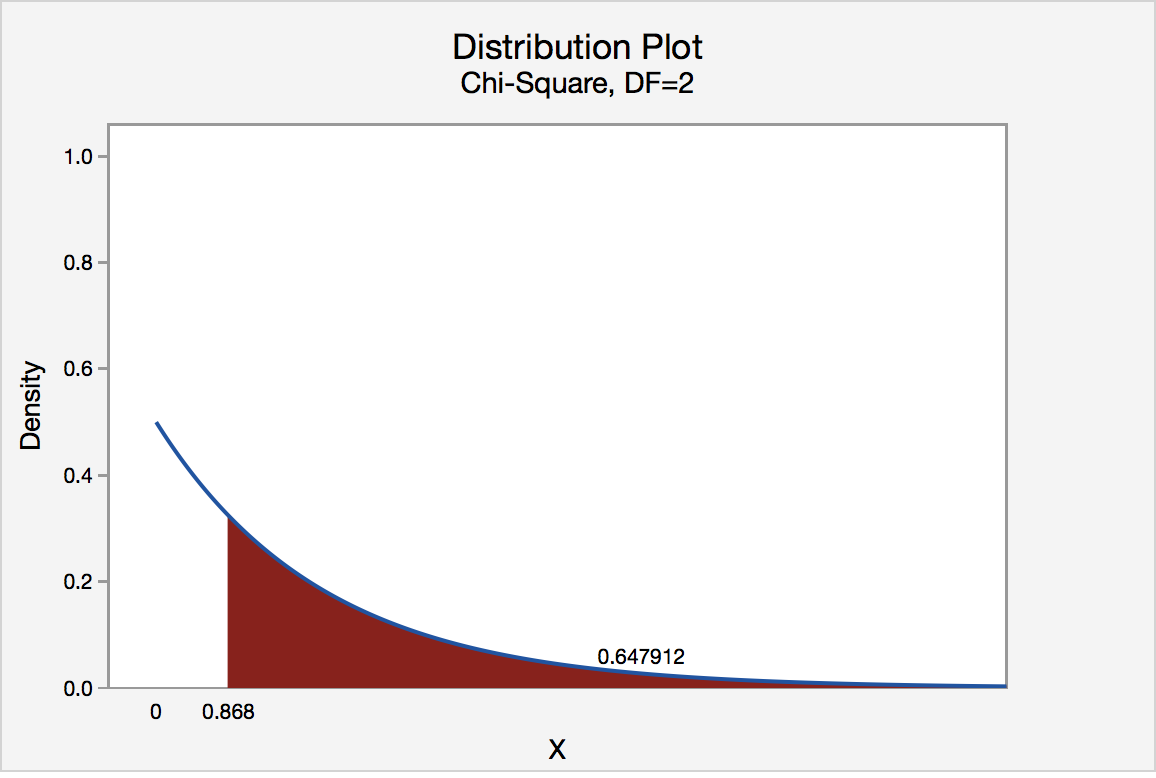# 11.2 - Goodness of Fit Test

11.2 - Goodness of Fit Test

A chi-square goodness-of-fit test can be conducted when there is one categorical variable with more than two levels. If there are exactly two categories, then a one proportion z test may be conducted. The levels of that categorical variable must be mutually exclusive. In other words, each case must fit into one and only one category.

We can test that the proportions are all equal to one another or we can test any specific set of proportions.

If the expected counts, which we'll learn how to compute shortly, are all at least five, then the chi-square distribution may be used to approximate the sampling distribution. If any expected count is less than five, then a randomization test should be conducted.

Possible Research Questions
• According to one research study, about 90% of American adults are right-handed, 9% are left-handed, and 1% are ambidextrous. Are the proportions of Penn State students who are right-handed, left-handed, and ambidextrous different from these national values?
• A concessions stand sells blue, red, purple, and green freezer pops. They survey a sample of children and ask which of the four colors is their favorite. They want to know if the colors differ in popularity.
Test Statistic

In conducting a goodness-of-fit test, we compare observed counts to expected counts. Observed counts are the number of cases in the sample in each group. Expected counts are computed given that the null hypothesis is true; this is the number of cases we would expect to see in each cell if the null hypothesis were true.

Expected Cell Value
$$E=n (p_i)$$

$$n$$ is the total sample size
$$p_i$$ is the hypothesized proportion of the "ith" group

The observed and expected values are then used to compute the chi-square ($$\chi^2$$) test statistic.

Chi-Square ($$\chi^2$$) Test Statistic

$$\chi^2=\sum \dfrac{(Observed-Expected)^2}{Expected}$$

Approximating the Sampling Distribution

StatKey has the ability to conduct a randomization test for a goodness-of-fit test. There is an example of this in Section 7.1 of the Lock5 textbook.  If all expected values are at least five, then the sampling distribution can be approximated using a chi-square distribution.

Like the t distribution, the chi-square distribution varies depending on the degrees of freedom. Degrees of freedom for a chi-square goodness-of-fit test are equal to the number of groups minus 1. The distribution plot below compares the chi-square distributions with 2, 4, and 6 degrees of freedom.To find the p-value we find the area under the chi-square distribution to the right of our test statistic. A chi-square test is always right-tailed.

# 11.2.1 - Five Step Hypothesis Testing Procedure

11.2.1 - Five Step Hypothesis Testing Procedure

The examples on the following pages use the five step hypothesis testing procedure outlined below. This is the same procedure that we used to conduct a hypothesis test for a single mean, single proportion, difference in two means, and difference in two proportions.

Step 1: Check assumptions and write hypotheses

When conducting a chi-square goodness-of-fit test, it makes the most sense to write the hypotheses first. The hypotheses will depend on the research question. The null hypothesis will always contain the equalities and the alternative hypothesis will be that at least one population proportion is not as specified in the null.

In order to use the chi-square distribution to approximate the sampling distribution, all expected counts must be at least five.

Expected Count
$$E=np_i$$

Where $$n$$ is the total sample size and $$p_i$$ is the hypothesized population proportion in the "ith" group.

To check this assumption, compute all expected counts and confirm that each is at least five.

Step 2: Compute the test statistic

In Step 1 you already computed the expected counts. Use this formula to compute the chi-square test statistic:

Chi-Square Test Statistic
$$\chi^2=\sum \dfrac{(O-E)^2}{E}$$
Where $$O$$ is the observed count for each cell and $$E$$ is the expected count for each cell.
Step 3: Determine the p-value

Construct a chi-square distribution with degrees of freedom equal to the number of groups minus one. The p-value is the area under that distribution to the right of the test statistic that was computed in Step 2. You can find this area by constructing a probability distribution plot in Minitab.

Step 4: Make a decision

Unless otherwise stated, use the standard 0.05 alpha level.

$$p \leq \alpha$$ reject the null hypothesis.

$$p > \alpha$$ fail to reject the null hypothesis.

Step 5: State a real-world conclusion

Go back to the original research question and address it directly. If you rejected the null hypothesis, then there is evidence that at least one of the population proportions is not as stated in the null hypothesis. If you failed to reject the null hypothesis, then there is not enough evidence that any of the population proportions are different from what is stated in the null hypothesis.

# 11.2.1.1 - Video: Cupcakes (Equal Proportions)

11.2.1.1 - Video: Cupcakes (Equal Proportions)

# 11.2.1.2- Cards (Equal Proportions)

11.2.1.2- Cards (Equal Proportions)

## Research question

When randomly selecting a card from a deck with replacement, are we equally likely to select a heart, diamond, spade, and club?

I randomly selected a card from a standard deck 40 times with replacement. I pulled 13 hearts, 8 diamonds, 8 spades, and 11 clubs.

Let's use the five-step hypothesis testing procedure:

Step 1: Check assumptions and write hypotheses

$$H_0: p_h=p_d=p_s=p_c=0.25$$
$$H_a:$$ at least one $$p_i$$ is not as specified in the null

We can use the null hypothesis to check the assumption that all expected counts are at least 5.

$$Expected\;count=n (p_i)$$

All $$p_i$$ are 0.25. $$40(0.25)=10$$, thus this assumption is met and we can approximate the sampling distribution using the chi-square distribution.

Step 2: Compute the test statistic

$$\chi^2=\sum \dfrac{(Observed-Expected)^2}{Expected}$$

All expected values are 10. Our observed values were 13, 8, 8, and 11.

$$\chi^2=\dfrac{(13-10)^2}{10}+\dfrac{(8-10)^2}{10}+\dfrac{(8-10)^2}{10}+\dfrac{(11-10)^2}{10}$$
$$\chi^2=\dfrac{9}{10}+\dfrac{4}{10}+\dfrac{4}{10}+\dfrac{1}{10}$$
$$\chi^2=1.8$$

Step 3: Determine the p-value

Our sampling distribution will be a chi-square distribution.

$$df=k-1=4-1=3$$

We can find the p-value by constructing a chi-square distribution with 3 degrees of freedom to find the area to the right of $$\chi^2=1.8$$The p-value is 0.614935

Step 4: Make a decision

$$p>0.05$$ therefore we fail to reject the null hypothesis.

Step 5: State a "real world" conclusion

There is not enough evidence to state that the proportion of hearts, diamonds, spades, and clubs that are randomly drawn from this deck are different.

# 11.2.1.3 - Roulette Wheel (Different Proportions)

11.2.1.3 - Roulette Wheel (Different Proportions)

## Research Question

An American roulette wheel contains 38 slots: 18 red, 18 black, and 2 green.  A casino has purchased a new wheel and they want to know if there is any evidence that the wheel is unfair. They spin the wheel 100 times and it lands on red 44 times, black 49 times, and green 7 times.

Step 1: Check assumptions and write hypotheses

If the wheel is fair then $$p_{red}=\dfrac{18}{38}$$, $$p_{black}=\dfrac{18}{38}$$, and $$p_{green}=\dfrac{2}{38}$$.

All of these proportions combined equal 1.

$$H_0: p_{red}=\dfrac{18}{38},\;p_{black}=\dfrac{18}{38}\;and\;p_{green}=\dfrac{2}{38}$$

$$H_a: At\;least\;one\;p_i\;is \;not\;as\;specified\;in\;the\;null$$

In order to conduct a chi-square goodness of fit test all expected values must be at least 5.

For both red and black: $$Expected \;count=100(\dfrac{18}{38})=47.368$$

For green: $$Expected\;count=100(\dfrac{2}{38})=5.263$$

All expected counts are at least 5 so we can conduct a chi-square goodness of fit test.

Step 2: Compute the test statistic

$$\chi^2=\sum \dfrac{(Observed-Expected)^2}{Expected}$$

In the first step we computed the expected values for red and black to be 47.368 and for green to be 5.263.

$$\chi^2= \dfrac{(44-47.368)^2}{47.368}+\dfrac{(49-47.368)^2}{47.368}+\dfrac{(7-5.263)^2}{5.263}$$

$$\chi^2=0.239+0.056+0.573=0.868$$

Step 3: Determine the p-value

Our sampling distribution will be a chi-square distribution.

$$df=k-1=3-1=2$$

We can find the p-value by constructing a chi-square distribution with 2 degrees of freedom to find the area to the right of $$\chi^2=0.868$$The p-value is 0.647912

Step 4: Make a decision

$$p>0.05$$ therefore we should fail to reject the null hypothesis.

Step 5: State a "real world" conclusion

There is not enough evidence that this roulette wheel is unfair.

# 11.2.2 - Minitab: Goodness-of-Fit Test

11.2.2 - Minitab: Goodness-of-Fit Test

## Research Question:

When randomly selecting a card from a deck with replacement, are we equally likely to select a heart, diamond, spade, and club?

I randomly selected a card from a standard deck 40 times with replacement. I pulled 13 Hearts (), 8 Diamonds (), 8 Spades (♠), and 11 Clubs (♣).

## Minitab® – Conducting a Chi-Square Goodness-of-Fit Test

### Summarized Data, Equal Proportions

To perform a chi-square goodness-of-fit test in Minitab using summarized data we first need to enter the data into the worksheet. Below you can see that we have one column with the names of each group and one column with the observed counts for each group.

C1 C2 Hearts 13 Diamonds 8 Spades 8 Clubs 11
1. After entering the data, select Stat > Tables > Chi-Square Goodness of Fit Test (One Variable)
2. Double-click Count to enter it into the Observed Counts box
3. Double-click Suit to enter it into the Category names (optional) box
4. Click OK

This should result in the following output:

#### Chi-Square Goodness-of-Fit Test: Count

##### Observed and Expected Counts
Category Observed Test
Proportion
Expected Contribution
to Chi-Sq
Hearts 13 0.25 10 0.9
Diamonds 8 0.25 10 0.4
Clubs 11 0.25 10 0.1
##### Chi-Square Test
N DF Chi-Sq P-Value
40 3 1.8 0.615

All expected values are at least 5 so we can use the chi-square distribution to approximate the sampling distribution. Our results are $$\chi^2 (3) = 1.8$$. $$p = 0.615$$. Because our p-value is greater than the standard alpha level of 0.05, we fail to reject the null hypothesis. There is not enough evidence to conclude that the proportions are different in the population.

## Note!

The example above tested equal population proportions. Minitab also has the ability to conduct a chi-square goodness-of-fit test when the hypothesized population proportions are not all equal. To do this, you can choose to test specified proportions or to use proportions based on historical counts.

# 11.2.2.1 - Example: Summarized Data, Equal Proportions

11.2.2.1 - Example: Summarized Data, Equal Proportions

## Example: Tulips

A company selling tulip bulbs claims they have equal proportions of white, pink, and purple bulbs and that they fill customer orders by randomly selecting bulbs from the population of all of their bulbs.

You ordered 30 bulbs and received 16 white, 8 pink, and 6 purple.

Is there evidence the bulbs you received were not randomly selected from a population with an equal proportion of each color?

Use Minitab to conduct a hypothesis test to address this research question.

We'll go through each of the steps in the hypotheses test:

Step 1: Check assumptions and write hypotheses

$$H_0\colon p_{white}=p_{pink}=p_{purple}=\dfrac{1}{3}$$
$$H_a\colon$$ at least one $$p_i$$ is not $$\dfrac{1}{3}$$

We can use the null hypothesis to check the assumption that all expected counts are at least 5.

$$Expected\;count=n (p_i)$$

All $$p_i$$ are $$\frac{1}{3}$$. $$30(\frac{1}{3})=10$$, thus this assumption is met and we can approximate the sampling distribution using the chi-square distribution.

Step 2: Compute the test statistic

Let's use Minitab to calculate this.

First, enter the summarized data into a Minitab Worksheet.

C1 C2 White 16 Pink 8 Purple 6
1. After entering the data, select Stat > Tables > Chi-Square Goodness of Fit Test (One Variable)
2. Double-click Count to enter it into the Observed Counts box
3. Double-click Color to enter it into the Category names (optional) box
4. Click OK

This should result in the following output:

#### Chi-Square Goodness-of-Fit Test: Count

##### Observed and Expected Counts
Category Observed Test
Proportion
Expected Contribution
to Chi-Sq
White 16 0.333333 10 3.6
Pink 8 0.333333 10 0.4
Purple 6 0.333333 10 1.6
##### Chi-Square Test
N DF Chi-Sq P-Value
30 2 5.6 0.061

The test statistic is a Chi-Square of 5.6.

Step 3: Determine the p-value
The p-value from the output is 0.061.

Step 4: Make a decision

$$p>0.05$$ therefore we fail to reject the null hypothesis.

Step 5: State a "real world" conclusion

There is not evidence that your tulip bulbs were not randomly selected from a population with equal proportions of white, pink and purple.

# 11.2.2.2 - Example: Summarized Data, Different Proportions

11.2.2.2 - Example: Summarized Data, Different Proportions

## Example: Roulette

An American roulette wheel contains 38 slots: 18 red, 18 black, and 2 green. A casino has purchased a new wheel and they want to know if there is any evidence that the wheel is unfair. They spin the wheel 100 times and it lands on red 44 times, black 49 times, and green 7 times.

Use Minitab to conduct a hypothesis test to address this question.

We'll go through each of the steps in the hypotheses test:

Step 1: Check assumptions and write hypotheses

If the wheel is 'fair' then the probability of red and black are both 18/38 and the probability of green is 2/38.

$$H_0\colon p_{red}=\dfrac{18}{38}, p_{black}=\dfrac{18}{38}, p_{green}=\dfrac{2}{38}$$
$$H_a\colon$$ at least one $$p_i$$ is not as specified in the null

We can use the null hypothesis to check the assumption that all expected counts are at least 5.

$$Expected\;count=n (p_i)$$

With n = 100 we meet the assumptions needed to use Chi-square.

Step 2: Compute the test statistic

Let's use Minitab to calculate this.

First, enter the summarized data into a Minitab Worksheet.

C1 C2 Red 44 Black 49 Green 7
1. After entering the data, select Stat > Tables > Chi-Square Goodness of Fit Test (One Variable)
2. Double-click Count to enter it into the Observed Counts box
3. Double-click Color to enter it into the Category names (optional) box
4. For Test select Input constants
5. Select Proportions specified by historical counts (this is what we would expect if the null was true)
6. Enter 18/38 for Black, 2/38 for Green and 18/38 for Red
7. Click OK

This should result in the following output:

#### Chi-Square Goodness-of-Fit Test: Count

##### Observed and Expected Counts
Category Observed Historical Counts Test
Proportion
Expected Contribution
to Chi-Sq
Red 44 18 0.473684 47.3684 0.239532
Black 49 18 0.473684 47.3684 0.056199
Green 7 2 0.052632 5.2632 0.573158
##### Chi-Square Test
N DF Chi-Sq P-Value
100 2 0.868889 0.648

The test statistic is a Chi-Square of 0.87.

Step 3: Determine the p-value
The p-value from the output is 0.648.

Step 4: Make a decision

$$p>0.05$$ therefore we fail to reject the null hypothesis.

Step 5: State a "real world" conclusion

There is not enough evidence to state that this roulette wheel is unfair.

  Link ↥ Has Tooltip/Popover Toggleable Visibility